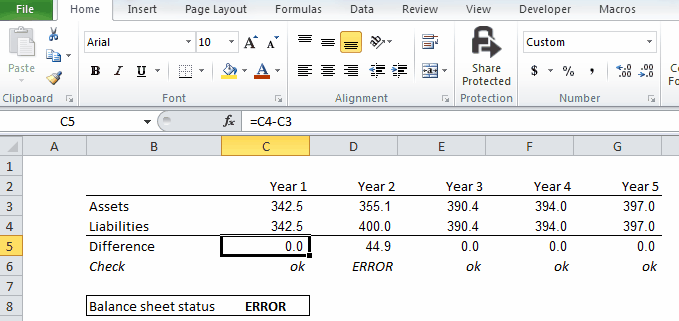top of page

## Coding

Checks

To make sure that your model works correctly and that there is no inconsistency or mistake, you should include as many checks as possible. One easy to creaste checks is to make sure that when a same result can be calculated through two differents ways the results is the same. However sometimes Excel takes the liberty of rounding numbers (after 10 decimals) and this can lead to having two slightly different result. In this case a simple formula checking if the two results are equal will not work as technically they are not. One way to overcome this issue is to include an acceptable margin of error. This can be done by calculating the absolute difference between the two results and making sure it is inferior to the specified acceptable margin of error.

Excel modeling

1. Calculate through two different ways the results that must be equal

2. Using the ABS and the If functions, create a formula that will check if the ABS(result #1 - Result #2)<0.01 (in this case the margin of error is 0.01) and that will return ok if the two results are equal and ERROR else

3. Create a formula using COUNTIF that will count the number of ERROR in the row (or column) where the checks are, if this number is superior to 0, then it should return ERROR, else okbottom of page